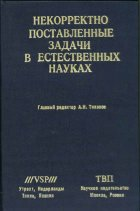Книги, изданные ТВП

Тихонов А. Н. (Гл. ред.)
Некорректно поставленные задачи в естественных науках
Труды Международной Московской конференции, 19-25 августа 1991 г.

Цена: 25.50 руб.Книга составлена из научных статей, представленных участниками Первой Международной конференции "Некорректно поставленные задачи в естественных науках", которая проходила в Москве с 19 по 25 августа 1991 года. Материал книги представляет интерес для специалистов в области прикладной математики, математической физики, теории некорректно поставленных и обратных задач, а также исследователей и инженеров, применяющих современные математические методы в акустике, астрофизике, геофизике, материаловедении, оптике, спектроскопии, томографии, электротехнике, энцефалографии, ядерной физике.

CONTENTS

Preface
Part I. THEORY AND METHODS OF SOLVING ILL-POSED PROBLEMS
Generalized maximum likelihood method and its application for solving ill-posed problems
 V.Ya.Arsenin
and A.V.Krianev
Iterative method for the solution of nonlinear ill-posed problems and their applications
A.B.Bakushinskii
Uniform regularization of first kind operator equations in Hilbert space
A.V.Cherepakhin
On the discretization error in regularized projection methods with parameter choice by discrepancy principle
U.Hämarik
Regularization and uniqueness of solutions of systems of Volterra nonlinear integral equations of the first kind with two arguments
M.I.Imanaliev and A.A.Asanov
Pointwise residual method for solving systems of linear algebraic equations and inequalities
A.Yu.Ivanitskii, F.P.Vasil'ev, and V.A.Morozov
Optimality region of the m-step Lavrentiev method
T.Kiho
Multilevel iterative methods for ill-posed problems
J.T.King
The method of minimal pseudoinversed matrix. Basic statements
A.S.Leonov
Variational algorithms with a posteriori choice of the regularization parameter for solving ill-posed extremal problems
A.S.Leonov
Tikhonov's approach for constructing regularizing algorithms
A.S.Leonov and A.G.Yagola
Regularization algorithms for solving ill-posed criterion problems
A.M.Levin
On the numerical solution of the ill-posed problems of construction of approximate solutions of singular integral equations of the second kind
A.F.Matveev
Regularization methods for ill-posed boundary problems
I.V.Mel'nikova
Modified Tikhonov regularization for nonlinear ill-posed problems leading to optimal convergence rates
A.Neubauer
On dynamical restoration of parameters of elliptic systems
Yu.S.Osipov and A.I.Korotkii
Extreme value estimation, a method for regularizing ill-posed inversion problems
P.Paatero
Two iterative schemes for solving linear non-necessarily well-posed problems in Banach spaces
R.Plato
On the regularization parameter choice for ill-posed problems with a quasisolution
T.Raus
An operator method of regularization of nonlinear monotone ill-posed problems
I.Rjazanceva
On the numerical inversion of the Laplace transform with boundary constraints
G.Rodriguez and S.Seatzu
Regularization of difference schemes
A.A.Samarskii and P.N.Vabishchevich
Ill-posed problems of on-line conditionally optimal filtering
I.N.Sinitsyn
The estimation of the error of the regularization method for ill-posed problems with noninjective operator
V.P.Tanana and T.N.Rudakova
Some numerical methods of parameter identification in nonlinear models
O.Vaarmann
Identification of filtration coefficient
G.Vainikko
Ill-posed problems and iterative approximation of fixed points of pseudo-contractive mappings
V.V.Vasin
Part 2. INVERSE PROBLEMS IN MATHEMATICAL PHYSICS
Nonlinear inverse problems of acoustic potential
G.V.Alekseyev and A.Yu.Chebotarev
A measure theoretic approach for studying inverse problems of the heat equation and the wave equation
G.Anger
New methods and results in multidimentional inverse problems for kinetic equations
Yu.E.Anikonov
An inverse problem originating from magnetohydrodynamics. Some numerical experiments
E.Beretta, E.Fischer, and M.Vogelius
Inverse conductivity problem in the two-dimentional case
V.G.Cherednichenko and G.V.Veryovkina
Inverse problems for a system of nonlinear partial differential equations
A.M.Denisov
An inverse problem in three-dimensional linear thermovicoelasticity of Boltzmann type
M.Grasselli
An inverse boundary value problem for the equation of the plate in the Love-Kirchhoff theory
M.Ikehata
Inverse problems for the nonstationary kinetic transport equation
A.L.Ivankov
Optimization methods of solving inverse problems of geoelectrics
S.I.Kabanikhin and A.L.Karchevsky
On passage to the limit in the inverse problems for nondivergence parabolic equations
V.L.Kamynin
One method of solution of the inverse problems for layered media
M.M.Lavrent'ev, Jr.
Identification problems for integrodifferential equations
A.Lorenzi
The Fredholm solvability of inverse problems for abstract differential equations
D.G.Orlovskii
Regularized traces of singular differential operators of higher orders
A.S.Pechentsov
Inverse problems for evolution equations
A.I.Prilepko, A.B.Kostin, and I.V.Tikhonov
Inverse problems in mathematical physics
A.I.Prilepko, D.G.Orlovskii, and I.A.Vasin
Stability estimates for inverse problems of geoelectrics
V.G.Romanov
Inverse problems for elliptic differential operators
Inverse boundary value problems in viscous fluid dynamics
I.A.Vasin
On some inverse problems for the time-dependent transport equation
N.P.Volkov
Determination of constant parameters in some semilinear parabolic equations
M.Yamamoto
Part 3. APPLICATIONS
A numerical method for solving inverse problem of deep magnetotelluric quasi-layered media sounding
I.S.Barashkov and V.I.Dmitriev
On numerical methods of solving inverse problems of electrical prospecting
S.M.Bersenev
The solution stability and restrictions on the space scatterer spectrum in the two-dimensional monochromatic inverse scattering problem
V.A.Burov and O.D.Rumiantseva
Inverse problems of astrophysics
A.M.Cherepashchuk, A.V.Goncharskii, and A.G.Yagola
Methods of solving the ion exchange inverse problem in counter-current columns
A.M.Denisov, S.R.Tuikina, and A.V.Chanov
Numerical methods for optical thin films at the National Research Council of Canada
J.A.Dobrowolski
Inverse scattering and synthesis problems in the diffraction theory
Ju.A.Eremin and A.G.Sveshnikov
The construction of numerical algorithms for ill-posed problems with random errors in initial data
A.M.Fedotov
A unified approach to the creation of knowledge-based systems for identification of some classes of nonstationary processes
V.Ya.Galkin and V.A.Karpenko
Inverse problems in vibrational spectroscopy
I.V.Kochikov, G.M.Kuramshina, Yu.A.Pentin, and A.G.Yagola
Inverse problems in electroencephalography and their numerical solving
Yu.M.Koptelov and E.V.Zakharov
A numerical method of crack determination by the boundary integral equation method
N.Nishimura
Regularization method in nonstationary inverse scattering problem
L.Nizhnik and R.Romanenko
Numerical methods for inverse scattering problems in two-dimensional scalar field
K.Onishi
On the hypersingular first kind integral equations for problems of electromagnetic scattering from screen surfaces
E.V.Zakharov and I.V.Halejeva
List of Contributors
National Organizing Committee

1992, ISBN 5-85484-001-4, 608 с., на англ. яз.